Courses

# Signals & Systems - MCQ Test - 2

## 20 Questions MCQ Test GATE ECE (Electronics) 2022 Mock Test Series | Signals & Systems - MCQ Test - 2

Description
This mock test of Signals & Systems - MCQ Test - 2 for Electronics and Communication Engineering (ECE) helps you for every Electronics and Communication Engineering (ECE) entrance exam. This contains 20 Multiple Choice Questions for Electronics and Communication Engineering (ECE) Signals & Systems - MCQ Test - 2 (mcq) to study with solutions a complete question bank. The solved questions answers in this Signals & Systems - MCQ Test - 2 quiz give you a good mix of easy questions and tough questions. Electronics and Communication Engineering (ECE) students definitely take this Signals & Systems - MCQ Test - 2 exercise for a better result in the exam. You can find other Signals & Systems - MCQ Test - 2 extra questions, long questions & short questions for Electronics and Communication Engineering (ECE) on EduRev as well by searching above.
QUESTION: 1

### If relation between input x[n] and output y[n] of a system is given by y[n] - 1/2 y[n -1] = x[n] then the system is

Solution:

Simply lake Z transform from both sides:

Then H(z)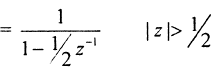it is right of the right - most pole

QUESTION: 2

### lf x[n] = - u[-n -1] + (1 /2)n u[-n -1] then ROC of signal is:

Solution: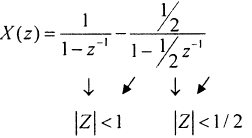Overall ROC is IZI < 1 /2

QUESTION: 3

### Consider, discrete time signal x[n] = (1 /3)n u[n] If signal y[n] is defined as y[n] = x[—n], —∞< n < ∞ then value of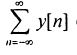equals to

Solution: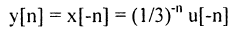Now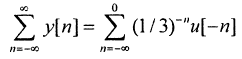Put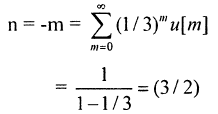QUESTION: 4

If signal x(t) and h(t) shown in figure are convolved to yield y(t).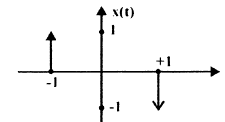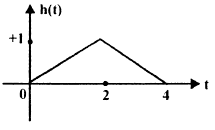Solution:

Simply use difference and sum between points.

QUESTION: 5

Given that g (t)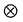g (t) = t exp (—t) u(t) then value of g(t) is

Solution: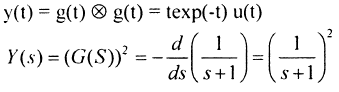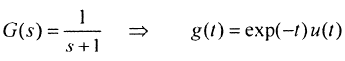QUESTION: 6

If u(t) r(t) denotes unit step and unit ramp respectively then u(t)r(t) denotes their convolution, then function u(t + 1)r(t — 2) is given by.

Solution:

y(t) = u(t)r(t)

Y(s) = 1/s3

QUESTION: 7

If wave form is given only for I period then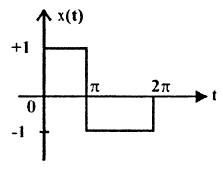Q. Waveform x (t) is

Solution:
QUESTION: 8

If wave form is given only for I period thenQ. Fourier series representation on of x (t) will be.

Solution:

it is an odd function with half symmetry.

QUESTION: 9

If complex Fourier- series coefficient Ck is given as Ck = (— 1/2)|k| then value of x(t) is:

Solution:
QUESTION: 10

Which statements are true about Fourier transform?

1. If x(t) is real then X(jω) is complex conjugate symmetric.
2. If x(t) is imaginary then X(jω) is complex conjugate anti-symmetric
3. If x(t) is even then X(jω) is also even.
Solution:
QUESTION: 11

Check status of linearity of given functions.

I .   x1(t) = x(t) .cost

2.  x2(t) = cos(x(t)).t

Solution:

x2(t) = t cos (x (t)

if x(t) = 0   ⇒  x2(t) = t

So x2(t) is non - linear

QUESTION: 12

If Fourier transform of x(t) is X(ω) then Fourier transform of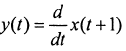Solution: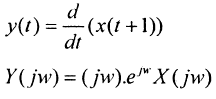QUESTION: 13

0.5 If Z-Transform X(z) of a sequence x[n] is given by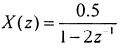.It is given that R.O.C. of X (z) includes unit circle then

Q. Value of x is

Solution: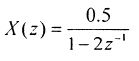QUESTION: 14

0.5 If Z-Transform X(z) of a sequence x[n] is given by.It is given that R.O.C. of X (z)includes unit circle then

Q. In above system if x[n] is causal then value of x is

Solution:

if it includes, unit - circle then, Iz| < 2

x[n] = - (0.5) 2n u(-n-1)

x = 0

if it is causal then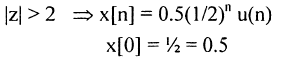QUESTION: 15

If frequency response of a causal & stable linear Time Invariant system is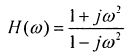, then value of phase delay and group delay are.

Solution: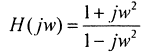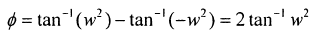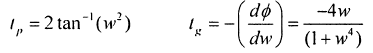QUESTION: 16

Which among the following are the stable discrete time systems?

1. y(n) = x(4n)
2. y(n) = x(-n)
3. y(n) = ax (n) + 8
4. y(n) = cos x(n)

Solution:
*Answer can only contain numeric values
QUESTION: 17

For given Fourier — series coefficients for real signals Co = 1, C1 = — 2, C2 = — 1 then power of this signal is _______

Solution:
QUESTION: 18

What is inverse Fourier transform of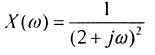Solution: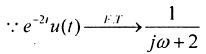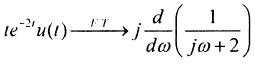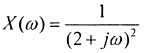QUESTION: 19

Which statement is true about y[n] = x[n] . cos (ωo n)

Solution:
*Answer can only contain numeric values
QUESTION: 20

For given signal s(t), shown below what is slope of matched filter output during interval 1 ≤ t ≤ 2 is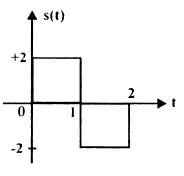Solution:

h(t) =    = s(2

y(t) = NWh(t )

Just draw y(t) and then calculate slope of y(t)